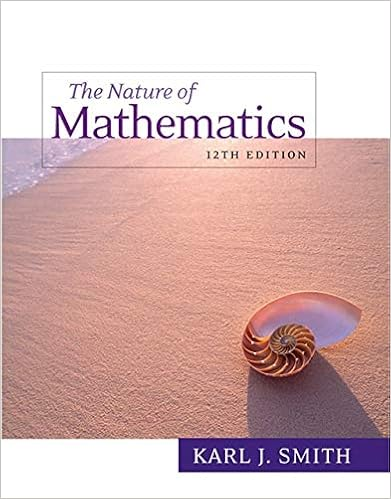The average weight of 6 men decreases by 3 kg when

• 5

This preview shows page 3 - 5 out of 5 pages.

We have textbook solutions for you!
The document you are viewing contains questions related to this textbook.The document you are viewing contains questions related to this textbook.
Chapter 5 / Exercise 5
Nature of Mathematics
SmithExpert Verified
15 The average weight of 6 men decreases by 3 kg when one of them weighing 80 kg is replaced by a new man. The weight of the new man is: a 62 b c 92 d 56 80
16 A library has an average of 510 visitors on Sundays and 240 on other days. The average number of visitors per day in a month of 30 days beginning with a Sunday is: 300 290 17 A pupil's marks were wrongly entered as 83 instead of 63. Due to that the average marks for
the class got increased by half (1/2). The number of pupils in the class is: 10 52 18 The average age of boys in the class is twice the number of girls in the class. If the ratio of
boys and girls in the class of 36 be 5 : 1, what is the total of the ages (in years) of the boys in the class. 196 360
19 The mean of 100 observations was calculated as 40. It was found later on that one of the observations was misread as 83 instead of 53. The correct mean is a 41 b 39.5 c 40.5 d 20 The average age of a class is 15.8 years. The average age of the boys in the class is 16.4 yrs while that of the girls is 15.4 years. What is the ratio of boys to girls in the class? . 40 a 2:3 b c 1:2 d 3:5 2:5
21 The average salary per month of 30 employees in a company is Rs 4000. If the manager’s salary is added, the average salary increases to Rs 4300, what is the salary of the manager:
We have textbook solutions for you!
The document you are viewing contains questions related to this textbook.The document you are viewing contains questions related to this textbook.
Chapter 5 / Exercise 5
Nature of Mathematics
SmithExpert Verified
22 Three years ago, the average age of a family of 5 members was 17 years. A baby having been born, the average age of the family is the same today . The present age of the baby is: 3 3.5
23 Of the three numbers, second is twice the first and is also thrice the third. If the average of the three numbers is 44, the largest number is: 24 108
24 Five years ago, the average age of A, B, C and D was 45 years. With E joining them now, the average of all the five is 49 years. How old is E? a 29 b c 54 d 60 45
25 The average age of husband, wife and their child 3 years ago was 27 years and that of wife and the child 5 years ago was 20 years. The present age of the husband is: 26 The captain of a cricket team of 11 members is 26 years old and the wicket keeper is 3 years
older. If the ages of these two are excluded, the average age of the remaining players is one year less than the average age of the whole team. What is the average age of the team?
•••